# 7.2 Right triangle trigonometry  (Page 6/12)

 Page 6 / 12

$\mathrm{sin}\text{\hspace{0.17em}}B=\frac{1}{\sqrt{3}},a=2$

$a=5,\measuredangle \text{\hspace{0.17em}}A=60°$

$b=\frac{5\sqrt{3}}{3},c=\frac{10\sqrt{3}}{3}$

$c=12,\measuredangle \text{\hspace{0.17em}}A=45°$

## Graphical

For the following exercises, use [link] to evaluate each trigonometric function of angle $\text{\hspace{0.17em}}A.$

$\mathrm{sin}\text{\hspace{0.17em}}A$

$\frac{5\sqrt{29}}{29}$

$\mathrm{cos}\text{\hspace{0.17em}}A$

$\mathrm{tan}\text{\hspace{0.17em}}A$

$\frac{5}{2}$

$\mathrm{csc}\text{\hspace{0.17em}}A$

$\mathrm{sec}\text{\hspace{0.17em}}A$

$\frac{\sqrt{29}}{2}$

$\mathrm{cot}\text{\hspace{0.17em}}A$

For the following exercises, use [link] to evaluate each trigonometric function of angle $\text{\hspace{0.17em}}A.$

$\mathrm{sin}\text{\hspace{0.17em}}A$

$\frac{5\sqrt{41}}{41}$

$\mathrm{cos}\text{\hspace{0.17em}}A$

$\mathrm{tan}\text{\hspace{0.17em}}A$

$\frac{5}{4}$

$\mathrm{csc}\text{\hspace{0.17em}}A$

$\mathrm{sec}\text{\hspace{0.17em}}A$

$\frac{\sqrt{41}}{4}$

$\mathrm{cot}\text{\hspace{0.17em}}A$

For the following exercises, solve for the unknown sides of the given triangle.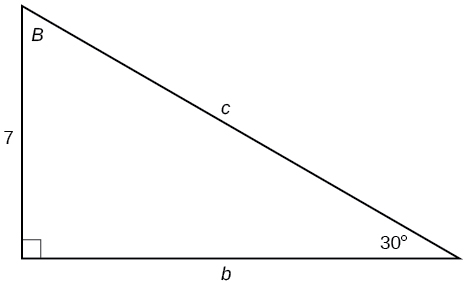$c=14,b=7\sqrt{3}$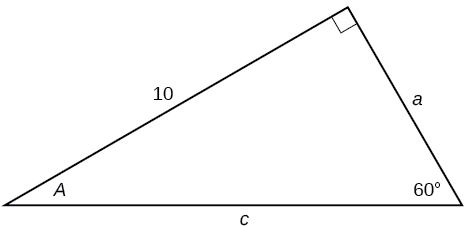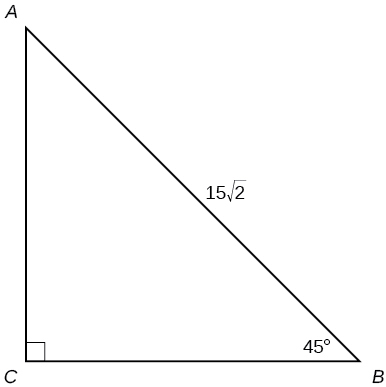$a=15,b=15$

## Technology

For the following exercises, use a calculator to find the length of each side to four decimal places.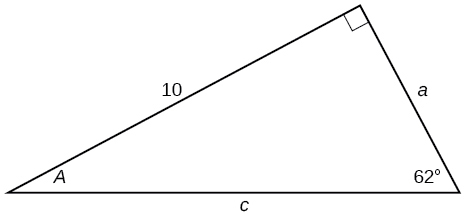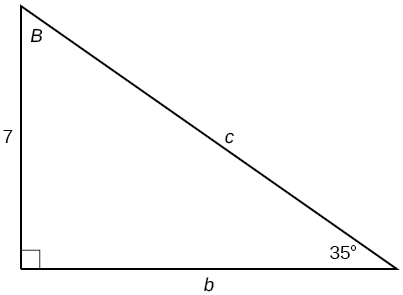$b=9.9970,c=12.2041$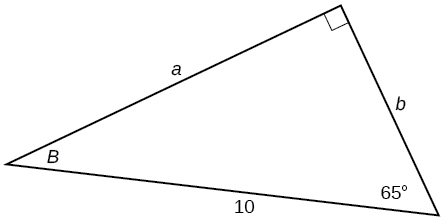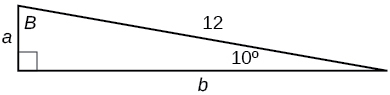$a=2.0838,b=11.8177$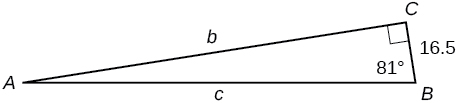$b=15,\measuredangle \text{\hspace{0.17em}}B=15°$

$a=55.9808,c=57.9555$

$c=200,\measuredangle \text{\hspace{0.17em}}B=5°$

$c=50,\measuredangle \text{\hspace{0.17em}}B=21°$

$a=46.6790,b=17.9184$

$a=30,\measuredangle \text{\hspace{0.17em}}A=27°$

$b=3.5,\measuredangle \text{\hspace{0.17em}}A=78°$

$a=16.4662,c=16.8341$

## Extensions

Find $\text{\hspace{0.17em}}x.$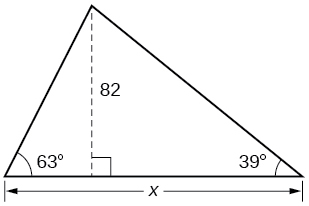Find $\text{\hspace{0.17em}}x.$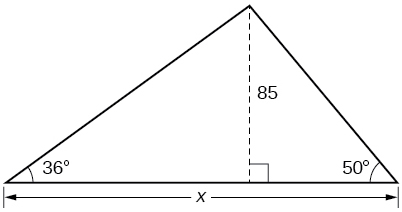188.3159

Find $\text{\hspace{0.17em}}x.$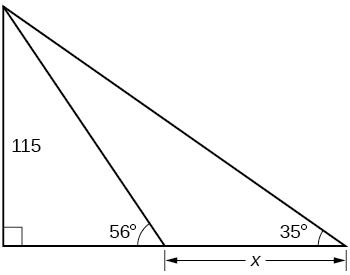Find $\text{\hspace{0.17em}}x.$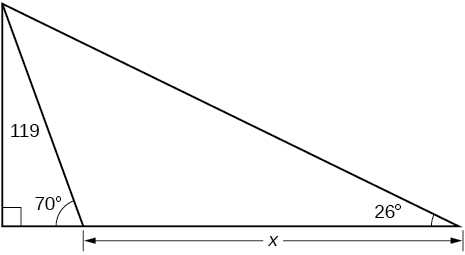200.6737

A radio tower is located 400 feet from a building. From a window in the building, a person determines that the angle of elevation to the top of the tower is $\text{\hspace{0.17em}}36°,$ and that the angle of depression to the bottom of the tower is $\text{\hspace{0.17em}}23°.\text{\hspace{0.17em}}$ How tall is the tower?

A radio tower is located 325 feet from a building. From a window in the building, a person determines that the angle of elevation to the top of the tower is $\text{\hspace{0.17em}}43°,$ and that the angle of depression to the bottom of the tower is $\text{\hspace{0.17em}}31°.\text{\hspace{0.17em}}$ How tall is the tower?

498.3471 ft

A 200-foot tall monument is located in the distance. From a window in a building, a person determines that the angle of elevation to the top of the monument is $\text{\hspace{0.17em}}15°,$ and that the angle of depression to the bottom of the monument is $\text{\hspace{0.17em}}2°.\text{\hspace{0.17em}}$ How far is the person from the monument?

A 400-foot tall monument is located in the distance. From a window in a building, a person determines that the angle of elevation to the top of the monument is $\text{\hspace{0.17em}}18°,$ and that the angle of depression to the bottom of the monument is $\text{\hspace{0.17em}}3°.\text{\hspace{0.17em}}$ How far is the person from the monument?

1060.09 ft

There is an antenna on the top of a building. From a location 300 feet from the base of the building, the angle of elevation to the top of the building is measured to be $\text{\hspace{0.17em}}40°.\text{\hspace{0.17em}}$ From the same location, the angle of elevation to the top of the antenna is measured to be $\text{\hspace{0.17em}}43°.\text{\hspace{0.17em}}$ Find the height of the antenna.

There is lightning rod on the top of a building. From a location 500 feet from the base of the building, the angle of elevation to the top of the building is measured to be $\text{\hspace{0.17em}}36°.\text{\hspace{0.17em}}$ From the same location, the angle of elevation to the top of the lightning rod is measured to be $\text{\hspace{0.17em}}38°.\text{\hspace{0.17em}}$ Find the height of the lightning rod.

27.372 ft

## Real-world applications

A 33-ft ladder leans against a building so that the angle between the ground and the ladder is $\text{\hspace{0.17em}}80°.\text{\hspace{0.17em}}$ How high does the ladder reach up the side of the building?

A 23-ft ladder leans against a building so that the angle between the ground and the ladder is $\text{\hspace{0.17em}}80°.\text{\hspace{0.17em}}$ How high does the ladder reach up the side of the building?

22.6506 ft

The angle of elevation to the top of a building in New York is found to be 9 degrees from the ground at a distance of 1 mile from the base of the building. Using this information, find the height of the building.

The angle of elevation to the top of a building in Seattle is found to be 2 degrees from the ground at a distance of 2 miles from the base of the building. Using this information, find the height of the building.

368.7633 ft

Assuming that a 370-foot tall giant redwood grows vertically, if I walk a certain distance from the tree and measure the angle of elevation to the top of the tree to be $\text{\hspace{0.17em}}60°,$ how far from the base of the tree am I?

#### Questions & Answers

write down the polynomial function with root 1/3,2,-3 with solution
if A and B are subspaces of V prove that (A+B)/B=A/(A-B)
write down the value of each of the following in surd form a)cos(-65°) b)sin(-180°)c)tan(225°)d)tan(135°)
Prove that (sinA/1-cosA - 1-cosA/sinA) (cosA/1-sinA - 1-sinA/cosA) = 4
what is the answer to dividing negative index
In a triangle ABC prove that. (b+c)cosA+(c+a)cosB+(a+b)cisC=a+b+c.
give me the waec 2019 questions
the polar co-ordinate of the point (-1, -1)
prove the identites sin x ( 1+ tan x )+ cos x ( 1+ cot x )= sec x + cosec x
tanh`(x-iy) =A+iB, find A and B
B=Ai-itan(hx-hiy)
Rukmini
what is the addition of 101011 with 101010
If those numbers are binary, it's 1010101. If they are base 10, it's 202021.
Jack
extra power 4 minus 5 x cube + 7 x square minus 5 x + 1 equal to zero
the gradient function of a curve is 2x+4 and the curve passes through point (1,4) find the equation of the curve
1+cos²A/cos²A=2cosec²A-1
test for convergence the series 1+x/2+2!/9x3ByByBy